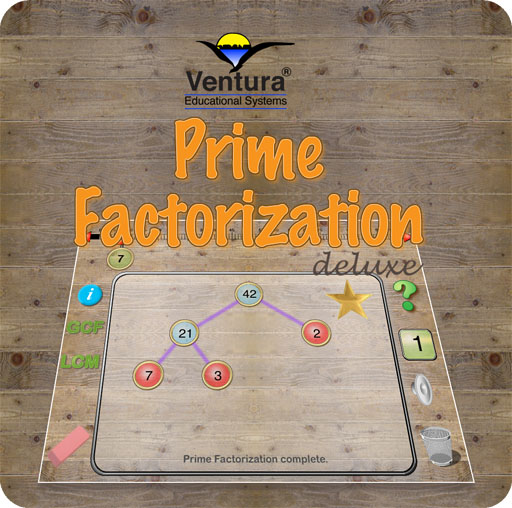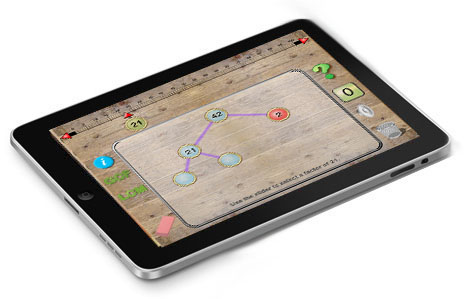Discover Prime Factorization, LCM and GCF with this interactive app!Interactive Excitement and a Great Learning Experience
 Use Prime Factorization Deluxe to explore and learn how to find the prime factorization of a composite number. Use the slider to select a number up to 100 for factorization. If a composite number is selected the app prompts the user to select a factor using the slider. If a prime is selected it is indicated using a red marker. Tap the question mark for a random number up to 100 to be automatically selected. Choose factors for the given number to build a factor tree. Continue until the prime factorization has been found. Learn how to find the greatest commonf factor (GCF) and least common multiple (LCM). For GCF, use the number line slider to select two numbers. The factors of the two numbers are show in a table and the greatest common factor is highlighted. For LCM, choose two numbers. The multiples of the number are shown in a table. The least common multiple is highlighted in the table. The first step in the process of prime factorization is to select a factor for the given number. If a factor is selected a branch on the tree is filled in. This process continues until all the composite numbers in the tree have been factored leaving only primes. Composite numbers are shown in blue and primes are highlighted in red. By examing the prime factorizations of two numbers students will notice that the Greatest Common Factor of two numbers is precisely the product of the primes that are common to both. Therefore it follows that the common multiples of any two numbers must contain each of the primes exactly once and that the least common multiple is the smallest number occurs in both sets.Special features make using Prime Factorization Deluxe fun: 1. Slide a marker to select factors. 2. Random problems are automatically generated. 3. Optional sound effects. 4. Available for iPad iOS 7 and 8.\$1.99